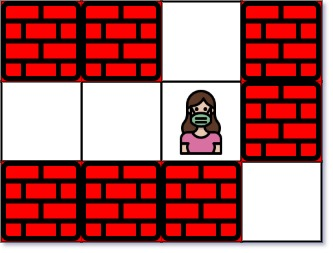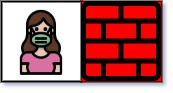## C# || Contains Duplicate II – How To Determine Two Distinct Indices In Array Equal To Target Value Using C#The following is a module with functions which demonstrates how to determine two distinct indices in array equal to target value using C#.

1. Contains Nearby Duplicate – Problem Statement

Given an integer array nums and an integer k, return true if there are two distinct indices i and j in the array such that nums[i] == nums[j] and abs(i – j) <= k.

Example 1:

``` Input: nums = [1,2,3,1], k = 3 Output: true ```

Example 2:

``` Input: nums = [1,0,1,1], k = 1 Output: true ```

Example 3:

``` Input: nums = [1,2,3,1,2,3], k = 2 Output: false ```

2. Contains Nearby Duplicate – Solution

The following are two solutions which demonstrates how to determine two distinct indices in array equal to target value.

``` 2. Contains Nearby Duplicate - Solution C# // ============================================================================ // Author: Kenneth Perkins // Date: Dec 25, 2022 // Taken From: http://programmingnotes.org/ // File: Solution.cs // Description: Demonstrates how to determine two indices equal to target value // ============================================================================ public class Solution { public bool ContainsNearbyDuplicate(int[] nums, int k) { var set = new HashSet<int>(); for (int index = 0; index < nums.Length; ++index){ if (index > k) { set.Remove(nums[index - k - 1]); } if (!set.Add(nums[index])) { return true; } } return false; } }// http://programmingnotes.org/ 123456789101112131415161718192021 // ============================================================================//    Author: Kenneth Perkins//    Date:   Dec 25, 2022//    Taken From: http://programmingnotes.org///    File:  Solution.cs//    Description: Demonstrates how to determine two indices equal to target value// ============================================================================public class Solution {    public bool ContainsNearbyDuplicate(int[] nums, int k) {        var set = new HashSet<int>();        for (int index = 0; index < nums.Length; ++index){            if (index > k) {                set.Remove(nums[index - k - 1]);            }            if (!set.Add(nums[index])) {                return true;            }        }        return false;    }}// http://programmingnotes.org/ ```

QUICK NOTES:
The highlighted lines are sections of interest to look out for.

The code is heavily commented, so no further insight is necessary. If you have any questions, feel free to leave a comment below.

Once compiled, you should get this as your output for the example cases:

``` true true false ```

## C# || How To Find The Nearest Exit From Entrance In Maze Using C#The following is a module with functions which demonstrates how to find the nearest exit from the entrance in a maze using C#.

1. Nearest Exit – Problem Statement

You are given an m x n matrix maze (0-indexed) with empty cells (represented as ‘.’) and walls (represented as ‘+’). You are also given the entrance of the maze, where entrance = [entrancerow, entrancecol] denotes the row and column of the cell you are initially standing at.

In one step, you can move one cell up, down, left, or right. You cannot step into a cell with a wall, and you cannot step outside the maze. Your goal is to find the nearest exit from the entrance. An exit is defined as an empty cell that is at the border of the maze. The entrance does not count as an exit.

Return the number of steps in the shortest path from the entrance to the nearest exit, or -1 if no such path exists.

Example 1:``` Input: maze = [["+","+",".","+"],[".",".",".","+"],["+","+","+","."]], entrance = [1,2] Output: 1 Explanation: There are 3 exits in this maze at [1,0], [0,2], and [2,3]. Initially, you are at the entrance cell [1,2]. - You can reach [1,0] by moving 2 steps left. - You can reach [0,2] by moving 1 step up. It is impossible to reach [2,3] from the entrance. Thus, the nearest exit is [0,2], which is 1 step away. ```

Example 2:``` Input: maze = [["+","+","+"],[".",".","."],["+","+","+"]], entrance = [1,0] Output: 2 Explanation: There is 1 exit in this maze at [1,2]. [1,0] does not count as an exit since it is the entrance cell. Initially, you are at the entrance cell [1,0]. - You can reach [1,2] by moving 2 steps right. Thus, the nearest exit is [1,2], which is 2 steps away. ```

Example 3:``` Input: maze = [[".","+"]], entrance = [0,0] Output: -1 Explanation: There are no exits in this maze. ```

2. Nearest Exit – Solution

The following is a solution which demonstrates how find the nearest exit from the entrance in a maze.

``` 2. Nearest Exit - Solution C# // ============================================================================ // Author: Kenneth Perkins // Date: Dec 1, 2022 // Taken From: http://programmingnotes.org/ // File: Solution.cs // Description: Demonstrates how to find nearest exit from entrance in maze // ============================================================================ public class Solution { public int NearestExit(char[][] maze, int[] entrance) { int rows = maze.Length; int cols = maze.Length; var directions = new List<KeyValuePair<int, int>>() { // Direction coordinates: (y, x) new KeyValuePair<int, int>(1, 0), new KeyValuePair<int, int>(-1, 0), new KeyValuePair<int, int>(0, 1), new KeyValuePair<int, int>(0, -1) }; char VISITED = '+'; char NOT_VISITED = '.'; // Mark the entrance as visited since its not a exit. int startRow = entrance; int startCol = entrance; maze[startRow][startCol] = VISITED; // Start BFS from the entrance, and use a queue `queue` to store all // the cells to be visited. var queue = new Queue<int[]>(); queue.Enqueue(new int[]{startRow, startCol, 0}); while (queue.Count > 0) { int[] currState = queue.Dequeue(); int currRow = currState; int currCol = currState; int currDistance = currState; // For the current cell, check its four neighbor cells. foreach (var dir in directions) { int nextRow = currRow + dir.Key; int nextCol = currCol + dir.Value; // If there exists an unvisited empty neighbor: if (IsValidCell(maze, nextRow, nextCol) && maze[nextRow][nextCol] == NOT_VISITED) { // If this empty cell is an exit, return distance + 1. if (nextRow == 0 || nextRow == rows - 1 || nextCol == 0 || nextCol == cols - 1) { return currDistance + 1; } // Otherwise, add this cell to 'queue' and mark it as visited. maze[nextRow][nextCol] = VISITED; queue.Enqueue(new int[]{nextRow, nextCol, currDistance + 1}); } } } // If we finish iterating without finding an exit, return -1. return -1; } private bool IsValidCell(char[][] grid, int row, int col) { return row >= 0 && row < grid.Length && col >= 0 && col < grid[row].Length; } }// http://programmingnotes.org/ 12345678910111213141516171819202122232425262728293031323334353637383940414243444546474849505152535455565758596061626364 // ============================================================================//    Author: Kenneth Perkins//    Date:   Dec 1, 2022//    Taken From: http://programmingnotes.org///    File:  Solution.cs//    Description: Demonstrates how to find nearest exit from entrance in maze// ============================================================================public class Solution {    public int NearestExit(char[][] maze, int[] entrance) {        int rows = maze.Length;        int cols = maze.Length;        var directions = new List<KeyValuePair<int, int>>() {            // Direction coordinates: (y, x)            new KeyValuePair<int, int>(1, 0),            new KeyValuePair<int, int>(-1, 0),            new KeyValuePair<int, int>(0, 1),            new KeyValuePair<int, int>(0, -1)        };        char VISITED = '+';        char NOT_VISITED = '.';         // Mark the entrance as visited since its not a exit.        int startRow = entrance;        int startCol = entrance;        maze[startRow][startCol] = VISITED;         // Start BFS from the entrance, and use a queue `queue` to store all        // the cells to be visited.        var queue = new Queue<int[]>();        queue.Enqueue(new int[]{startRow, startCol, 0});         while (queue.Count > 0) {            int[] currState = queue.Dequeue();            int currRow = currState;            int currCol = currState;            int currDistance = currState;             // For the current cell, check its four neighbor cells.            foreach (var dir in directions) {                int nextRow = currRow + dir.Key;                int nextCol = currCol + dir.Value;                 // If there exists an unvisited empty neighbor:                if (IsValidCell(maze, nextRow, nextCol) && maze[nextRow][nextCol] == NOT_VISITED) {                    // If this empty cell is an exit, return distance + 1.                    if (nextRow == 0 || nextRow == rows - 1 || nextCol == 0 || nextCol == cols - 1) {                        return currDistance + 1;                    }                     // Otherwise, add this cell to 'queue' and mark it as visited.                    maze[nextRow][nextCol] = VISITED;                    queue.Enqueue(new int[]{nextRow, nextCol, currDistance + 1});                }            }        }         // If we finish iterating without finding an exit, return -1.        return -1;    }     private bool IsValidCell(char[][] grid, int row, int col) {        return row >= 0 && row < grid.Length && col >= 0 && col < grid[row].Length;    }}// http://programmingnotes.org/ ```

QUICK NOTES:
The highlighted lines are sections of interest to look out for.

The code is heavily commented, so no further insight is necessary. If you have any questions, feel free to leave a comment below.

Once compiled, you should get this as your output for the example cases:

``` 1 2 -1 ```